### 2. COSMOLOGY WITH A COSMOLOGICAL CONSTANT

2.1 Cosmological parameters

From the Friedmann equation (5) (where henceforth we take the effects of a cosmological constant into account by including the vacuum energy density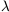into the total density), for any value of the Hubble parameter H there is a critical value of the energy density such that the spatial geometry is flat (k = 0):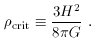(21)

It is often most convenient to measure the total energy density in terms of the critical density, by introducing the density parameter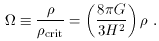(22)

One useful feature of this parameterization is a direct connection between the value ofand the spatial geometry:(23)

[Keep in mind that some references still use ``'' to refer strictly to the density parameter in matter, even in the presence of a cosmological constant; with this definition (23) no longer holds.]

In general, the energy densitywill include contributions from various distinct components. From the point of view of cosmology, the relevant feature of each component is how its energy density evolves as the universe expands. Fortunately, it is often (although not always) the case that individual components i have very simple equations of state of the form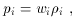(24)

with wi a constant. Plugging this equation of state into the energy-momentum conservation equationµ Tµ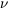= 0, we find that the energy density has a power-law dependence on the scale factor,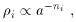(25)

where the exponent is related to the equation of state parameter by(26)

The density parameter in each component is defined in the obvious way,(27)

which has the useful property that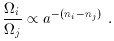(28)

The simplest example of a component of this form is a set of massive particles with negligible relative velocities, known in cosmology as ``dust'' or simply ``matter''. The energy density of such particles is given by their number density times their rest mass; as the universe expands, the number density is inversely proportional to the volume while the rest masses are constant, yieldingMa-3. For relativistic particles, known in cosmology as ``radiation'' (although any relativistic species counts, not only photons or even strictly massless particles), the energy density is the number density times the particle energy, and the latter is proportional to a-1 (redshifting as the universe expands); the radiation energy density therefore scales asRa-4. Vacuum energy does not change as the universe expands, soa0; from (26) this implies a negative pressure, or positive tension, when the vacuum energy is positive. Finally, for some purposes it is useful to pretend that the -ka-2 R0-2 term in (5) represents an effective ``energy density in curvature'', and define_k-(3k / 8GR02) a-2. We can define a corresponding density parameter(29)

this relation is simply (5) divided by H2. Note that the contribution fromk is (for obvious reasons) not included in the definition of. The usefulness ofk is that it contributes to the expansion rate analogously to the honest density parametersi; we can write(30)

where the notationi(k) reflects the fact that the sum includesk in addition to the various components of=ii. The most popular equations of state for cosmological energy sources can thus be summarized as follows: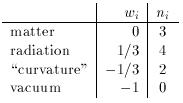(31)

The ranges of values of thei's which are allowed in principle (as opposed to constrained by observation) will depend on a complete theory of the matter fields, but lacking that we may still invoke energy conditions to get a handle on what constitutes sensible values. The most appropriate condition is the dominant energy condition (DEC), which states that Tµlµ l0, and Tµlµ is non-spacelike, for any null vector lµ; this implies that energy does not flow faster than the speed of light . For a perfect-fluid energy-momentum tensor of the form (4), these two requirements imply that+ p0 and |||p|, respectively. Thus, either the density is positive and greater in magnitude than the pressure, or the density is negative and equal in magnitude to a compensating positive pressure; in terms of the equation-of-state parameter w, we have either positiveand |w|1 or negativeand w = -1. That is, a negative energy density is allowed only if it is in the form of vacuum energy. (We have actually modified the conventional DEC somewhat, by using only null vectors lµ rather than null or timelike vectors; the traditional condition would rule out a negative cosmological constant, which there is no physical reason to do.)

There are good reasons to believe that the energy density in radiation today is much less than that in matter. Photons, which are readily detectable, contribute~ 5 x 10-5, mostly in the 2.73 °K cosmic microwave background [18, 19, 20]. If neutrinos are sufficiently low mass as to be relativistic today, conventional scenarios predict that they contribute approximately the same amount . In the absence of sources which are even more exotic, it is therefore useful to parameterize the universe today by the values ofM and, withk = 1 -M -, keeping the possibility of surprises always in mind.

One way to characterize a specific Friedmann-Robertson-Walker model is by the values of the Hubble parameter and the various energy densitiesi. (Of course, reconstructing the history of such a universe also requires an understanding of the microphysical processes which can exchange energy between the different states.) It may be difficult, however, to directly measure the different contributions to, and it is therefore useful to consider extracting these quantities from the behavior of the scale factor as a function of time. A traditional measure of the evolution of the expansion rate is the deceleration parameter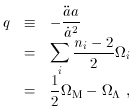(32)

where in the last line we have assumed that the universe is dominated by matter and the cosmological constant. Under the assumption that= 0, measuring q0 provides a direct measurement of the current density parameterM0; however, onceis admitted as a possibility there is no single parameter which characterizes various universes, and for most purposes it is more convenient to simply quote experimental results directly in terms ofM and. [Even this parameterization, of course, bears a certain theoretical bias which may not be justified; ultimately, the only unbiased method is to directly quote limits on a(t).]

Notice that positive-energy-density sources with n > 2 cause the universe to decelerate while n < 2 leads to acceleration; the more rapidly energy density redshifts away, the greater the tendency towards universal deceleration. An empty universe (= 0,k = 1) expands linearly with time; sometimes called the ``Milne universe'', such a spacetime is really flat Minkowski space in an unusual time-slicing.